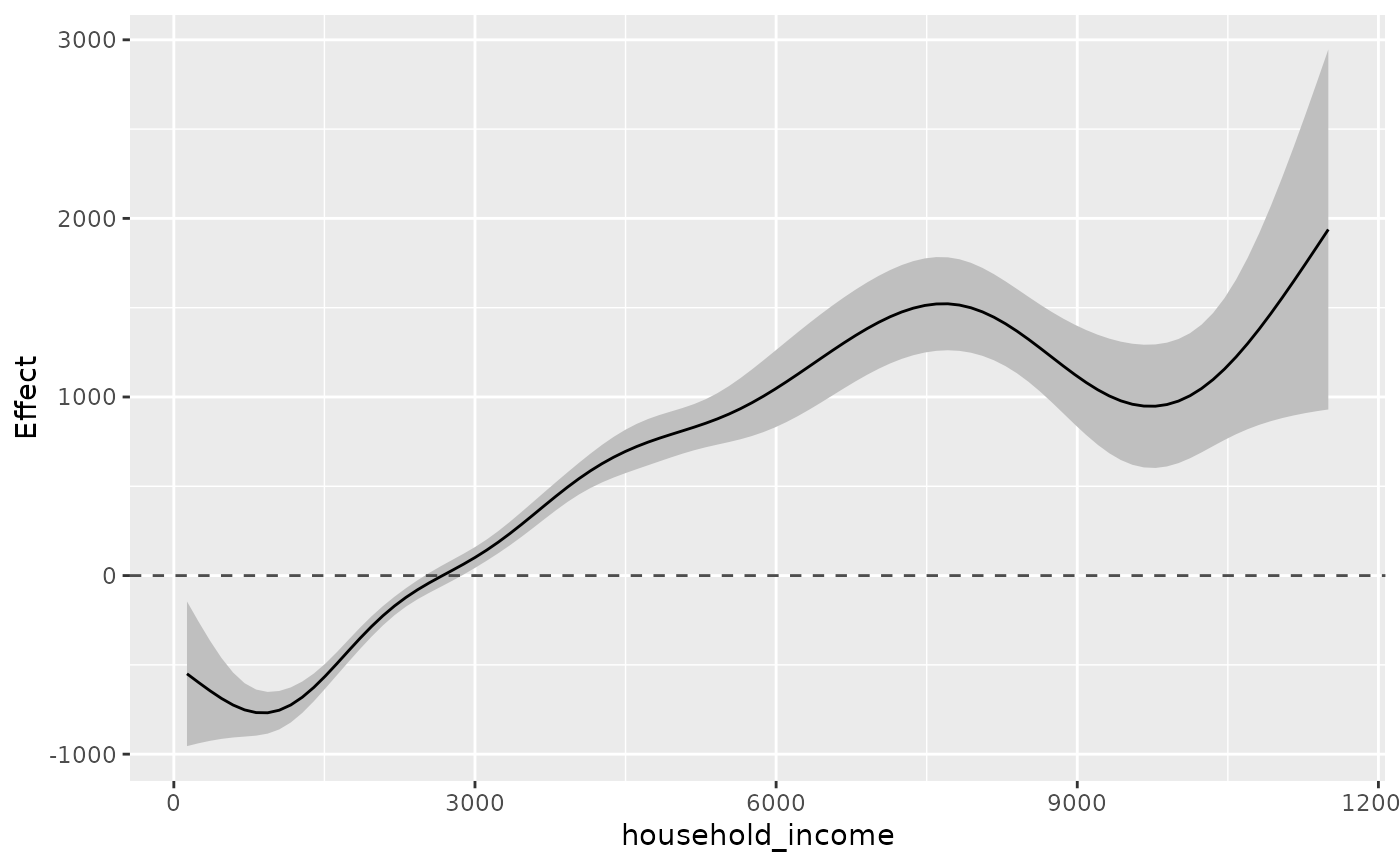Plots 1D smooth effects for a GAM model fitted with gam or bam.

## Usage

plot_1Dsmooth(
model,
plot_ci = TRUE,
select,
alpha = 0.05,
ylim = NULL,
method_expTransform = "simple",
return_plotData = FALSE
)

## Arguments

model

GAM model fitted with gam or bam.

plot_ci

If TRUE CIs are plotted. Only used if plot_type = 1.

select

Index of smooth term to be plotted.

alpha

(1-alpha) CIs are calculated. The default 0.05 leads to 95% CIs.

ylim

Optional limits of the y-axis.

method_expTransform

One of c("simple","delta"), stating if standard errors and confidence interval limits should be transformed by a simple exp transformation or using the delta method. The delta method can be unstable in situations and lead to negative confidence interval limits. Only used when the model was estimated with a log or logit link.

return_plotData

If TRUE, the dataset prepared for plotting is returned. Defaults to FALSE.

ggplot object

## Details

If the model was estimated with a log or logit link, the function automatically performs an exponential transformation of the effect, see argument method_expTransform.

## Author

Alexander Bauer alexander.bauer@stat.uni-muenchen.de

## Examples

library(APCtools)
library(mgcv)

data(travel)
model <- gam(mainTrip_distance ~ te(age, period) + residence_region +
household_size + s(household_income), data = travel)

plot_1Dsmooth(model, select = 2)